Next: Variance of the sample Up: TIME-STATISTICAL RESOLUTION Previous: Probability and independence

## Sample mean

Now let xt be a time series made up of identically distributed random numbers: mx and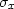do not depend on time. Let us also suppose that they are independently chosen; this means in particular that for any different times t and s ():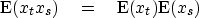(19)
Suppose we have a sample of n points of xt and are trying to determine the value of mx. We could make an estimate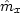of the mean mx with the formula(20)

A somewhat more elaborate method of estimating the mean would be to take a weighted average. Let wt define a set of weights normalized so that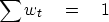(21)
With these weights, the more elaborate estimate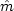of the mean is(22)
Actually (20) is just a special case of (22); in (20) the weights are wt = 1/n.

Further, the weights could be convolved on the random time series, to compute local averages of this time series, thus smoothing it. The weights are simply a filter response where the filter coefficients happen to be positive and cluster together. Figure 6 shows an example: a random walk function with itself smoothed locally.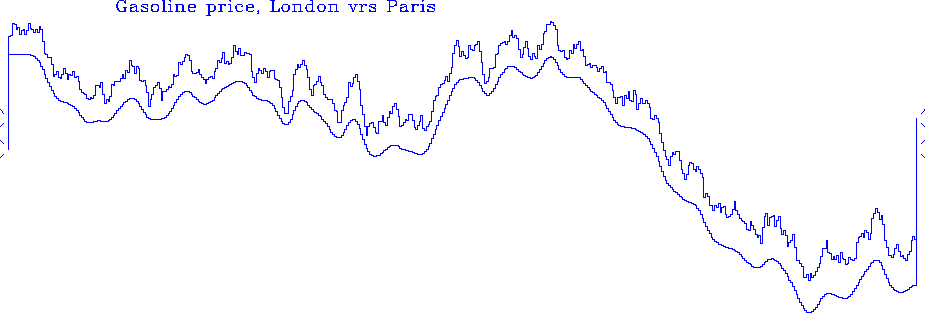walk
Figure 6
Random walk and itself smoothed (and shifted downward).Next: Variance of the sample Up: TIME-STATISTICAL RESOLUTION Previous: Probability and independence
Stanford Exploration Project
10/21/1998